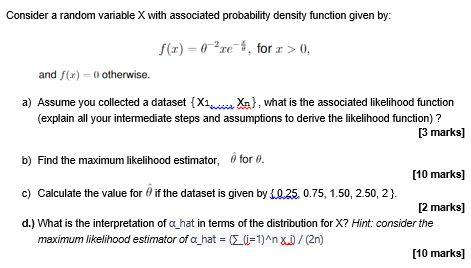Home / Expert Answers / Statistics and Probability / consider-a-random-variable-x-with-associated-probability-density-function-given-by-f-x-2xe-pa138

# (Solved): Consider a random variable X with associated probability density function given by: f(x)=2xe ...Consider a random variable with associated probability density function given by: and otherwise. a) Assume you collected a dataset , what is the associated likelihood function (explain all your intermediate steps and assumptions to derive the likelihood function)? [3 marks] b) Find the maximum likelihood estimator, for . [10 marks] c) Calculate the value for if the dataset is given by . [2 marks] d.) What is the interpretation of hat in terms of the distribution for ? Hint: consider the maximum likelihood estimator of hat [10 marks]

We have an Answer from Expert

a) To derive the likelihood function, we need to find the joint probability density function (pdf) for the dataset {X?, X?, ..., X?}. Since the random variable X has a probability density function given by:
f(x) = 0, otherwise The joint probability density function (pdf) can be expressed as the product of individual densities for each observation:    Substituting the given probability density function:    Taking the product and rearranging, we get:

Refer above solution

We have an Answer from Expert